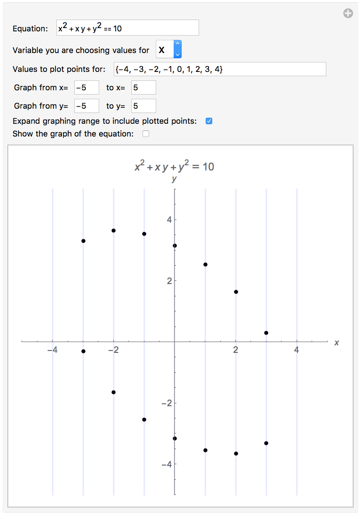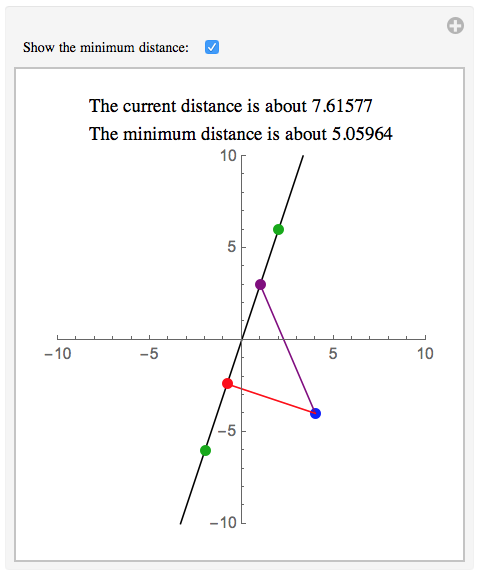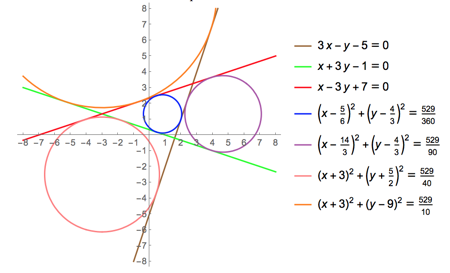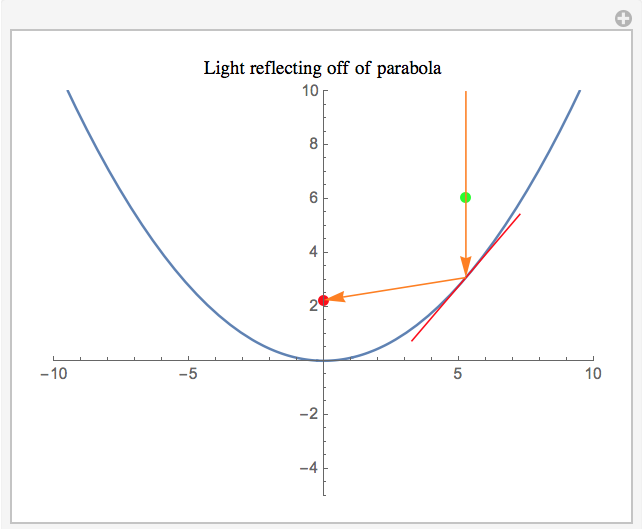# Analytic Geometry with Mathematica

It is becoming more difficult to find a textbook for Analytic Geometry.  The textbook we have used at Southeastern is long out of print, so Dr. Layne Heitz (who has taught Analytic for us the last few years) suggested that we write our own.  I thought it would be a great idea to write the text in Mathematica itself so that we could take advantage of all the algebra, graphing features, and interactive manipulations Mathematica provides.  So together we sat down and over the summer and fall of 2015 wrote up a working draft which you can download here and use for free.

Please note that updates were made to the text in the Spring and Summer of 2019 (last edits were on August 3rd 2019).  These updates include a range of corrections (mostly typographical and grammatical), the addition of some additional homework for Chapter 4, and the inclusion of some new optional material in Chapter 3 (showing how some of the problems can be done with geometry-oriented commands introduced in Mathematica 12).

You can find the following topics in the 4 Mathematica notebooks:

Chapter 1:  Distance, Points of Division, Slopes, Angles Between Lines, Graphs of Equations and Points of Intersection,  Equations of a LocusAn interactive grapher

Chapter 2: Equations of Lines, Distance from a Point to a Line, Families of Linesan interactive display of the distance from a point to a line

Chapter 3: Equations of Circles, Finding Circles that Satisfy Given Conditions, Tangent Lines, Families of Circlesthe circles tangent to 3 lines